# 5 Results

View
Selected filters:
• Number Patterns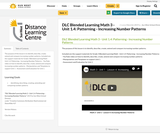Conditional Remix & Share Permitted
CC BY-NC-SA
Rating

The purpose of this lesson is to identify, describe, create, extend and compare increasing number patterns.

Included are the support materials for Grade 3 Blended Learning Math - Unit 1.4: Patterning - Increasing Number Patterns:
- YouTube video on how to identify, describe, create, extend and compare increasing number patterns

Subject:
Math
Material Type:
Activity/Lab
Homework/Assignment
Lesson
Provider:
Sun West Distance Learning Centre (DLC)
Author:
Sun West School Division
04/04/2019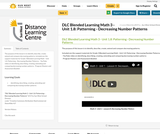Conditional Remix & Share Permitted
CC BY-NC-SA
Rating

The purpose of this lesson is to identify, describe, create, extend and compare decreasing patterns.

Included are the support materials for Grade 3 Blended Learning Math - Unit 1.8: Patterning - Decreasing Number Patterns:
- YouTube video on identifying, describing, creating, extending and comparing decreasing number patterns

Subject:
Math
Material Type:
Activity/Lab
Homework/Assignment
Lesson
Provider:
Sun West Distance Learning Centre (DLC)
Author:
Sun West School Division
04/04/2019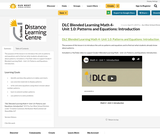Conditional Remix & Share Permitted
CC BY-NC-SA
Rating

The purpose of this lesson is to introduce the unit on patterns and equations and to find out what students already know about patterns.

Included is a YouTube video to support Grade 4 Blended Learning Math - Unit 1.0: Patterns and Equations: Introduction.

Subject:
Math
Material Type:
Activity/Lab
Homework/Assignment
Lesson
Provider:
Sun West Distance Learning Centre (DLC)
Author:
Sun West School Division
04/09/2019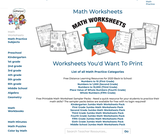Rating

"Free Printable Math Worksheet Sampler Packs - Need a quick resource for your students to practice their math skills? The sampler packs below are available for free with no login required!"

Grades K-9 + Algebra & Geometry.

Jumbo Math Worksheets Pack

Subject:
Math
Material Type:
Activity/Lab
Author:
edhelper
08/24/2020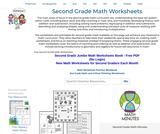Rating

The main areas of focus in the second grade math curriculum are: understanding the base-ten system within 1,000, including place value and skip-counting in fives, tens, and hundreds; developing fluency with addition and subtraction, including solving word problems; regrouping in addition and subtraction; describing and analyzing shapes; using and understanding standard units of measure; working with money and time; and introducing multiplication.

The worksheets and printables for second grade math available on this page will enhance any classroom's math curriculum. These engaging second grade math worksheets cover the basics of counting and ordering as well as addition and subtraction, and include exciting introductions to geometry and algebra for future self-assurance in math.

Subject:
Math
Material Type:
Activity/Lab
Assessment
Homework/Assignment
Teaching/Learning Strategy
Author:
edHelper